# GMAT Math : DSQ: Solving linear equations with one unknown

## Example Questions

2 Next →

### Example Question #11 : Linear Equations, One Unknown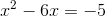. What is?

(1)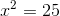(2)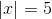Statement 2 alone is sufficient.

Statements 1 and 2 together are not sufficient.

Statement 1 alone is sufficient.

Both statements together are insufficient.

Each statement alone is sufficient.

Each statement alone is sufficient.

Explanation:

Firstly, we should try to find a simplified equation to better see the possible values for. We get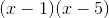. We can see thatcan either beor.

Statement 1 tells us that the square ofis. It follows thatmust be, therefore, this statement is sufficient.

Statement 2 tells us that the absolute value ofis, thereforemust beand therefore the statement is also sufficient alone.

Note that it is possible to answer with either statement only becausecan either beor. Ifcould have beenorthan statements 1 and 2 would have been insufficient.

### Example Question #931 : Data Sufficiency Questions

What isif,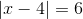?

(1)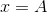(2)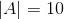Both statements together are sufficient.

Each statement alone is sufficient.

Statement 2 alone is sufficient.

Statement 1 alone is sufficient.

Statements 1 and 2 together are not sufficient.

Both statements together are sufficient.

Explanation:

To begin with this problem, we should try to solve. It gives us two sets of equations for us to find values for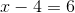and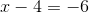. Solving gives us two possible values for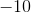and. Let's see how can the statements help us determine the value of.

Statement 1 gives us an other unknown for the value of. Therefore, this statement is insufficient.

Statement 2 gives an absolute value for this unknown. But we don't know what other values isequal to.

Taken together these statements, allow us to see thatmust beand therefore are sufficient to answer the question.

### Example Question #932 : Data Sufficiency Questions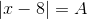. What is?

(1)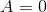(2)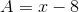Statements 1 and 2 together are not sufficient.

Statement 1 alone is sufficient.

Each statement alone is sufficient.

Both statements together are sufficient.

Statement 2 alone is sufficient.

Each statement alone is sufficient.

Explanation:

To approach this problem, we should firstly set up two possible equations for the value of; either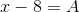or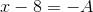Statement 1 tells us thatis in fact zero. Than the equations return a single value for, therefore statement 1 alone is sufficient.

Statement 2 tells us that, if plug in this value for, we get that: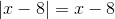Because there is the absolute value we get two equations: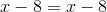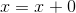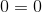or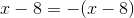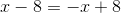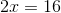must be 8. Plugging in the value forin our first equation gives us no solution because both sides are of equal value and we end up with.

Therefore, statement 2 alone is sufficient

To conclude, each statement alone is sufficient.

### Example Question #933 : Data Sufficiency Questions

What is?

(1)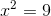(2)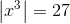Statement 2 alone is sufficient.

Each statement alone is suffcient.

Both statements together are sufficient.

Statements 1 and 2 taken together are not sufficient.

Statement 1 alone is sufficient.

Statements 1 and 2 taken together are not sufficient.

Explanation:

To answer this question, we must find a single value for.

Statement 1 gives us an equation with two possible solutions for. Therefore, statement 1 alone is not sufficient, sincecan either beorStatemnt 2 alone is also insufficient, because it gives us the same possible values foras the equation in statement 1.

When two statements give us the same the information the answer is either both statements together are sufficient or statements 1 and 2 together are not sufficient. Here neither statement allowed us to answer, it follows that statements 1 and 2 together are not sufficient.

### Example Question #934 : Data Sufficiency Questions, What is?

(1)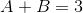(2)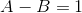Statement 2 alone is sufficient.

Statements 1 and 2 together are not sufficient.

Both statements together are sufficient.

Statement 1 alone is sufficient.

Each statement alone is sufficient.

Both statements together are sufficient.

Explanation:

To find a value for, we should be able to get a value for.

Statement 1 has two unknowns therefore we need another different equation with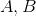to be able to find values for these unknowns.

Statement 2 alone is also insufficient because just as statement 1 has two variables and therefore we need more information to solve it.

Taking together these equations, by adding both sides we get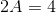and from there we can find a single value for.

Both statements together are sufficient.

### Example Question #935 : Data Sufficiency Questions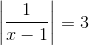andis different than. What is?

(1)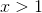(2)is not an integer.

Each statement alone is sufficient.

Both statements together are sufficient.

Statement 1 alone is sufficient.

Statement 2 alone is sufficient.

Statements 1 and 2 together are sufficient.

Statement 1 alone is sufficient.

Explanation:

Firstly we should try to see what are the possible values for, by solving the equations given by the absolute value:

either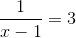or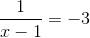.

This allows us to find two values forwhich areand, let's see how the statements can help us determine a single value for.

Statement 1 tells us thatmust be greater than one. Only one of our solutions foris greater than one. Therefore, statement 1 alone is sufficient.

Statement 2 tells us thatis not an integer, however both solutions are not integer values and therefore statement 2 doesn't help us find a single solution.

Statement 1 alone is sufficient.

2 Next →

Tired of practice problems?

Try live online GMAT prep today.